IMO Shortlist 2015 problem N8

Kvaliteta:
Avg: 0,0
Težina:
Avg: 9,0

For every positive integer$n$ with prime factorization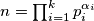$n = \prod_{i = 1}^{k} p_i^{\alpha_i}$, define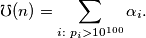That is,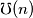$\mho(n)$ is the number of prime factors of$n$ greater than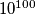$10^{100}$, counted with multiplicity.

Find all strictly increasing functions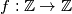$f: \mathbb{Z} \to \mathbb{Z}$ such that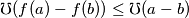for all integers$a$ and$b$ with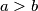$a > b$.

(Brazil)

Izvor: https://www.imo-official.org/problems/IMO2015SL.pdf